# Frank Solutions for Class 9 Maths Chapter 12 Isosceles triangle

Frank Solutions for Class 9 Maths Chapter 12 Isosceles Triangle provide comprehensive answers with the intention of helping students in solving the most tricky questions with ease. These solutions are helpful in clearing their doubts quickly while revising for the final examination. To obtain in-depth conceptual knowledge, students can practise Frank Solutions on a daily basis.

Chapter 12 consists of problems based on the Isosceles Triangle. Students who want to come out with flying colours in the annual exams are suggested to practise Frank Solutions regularly. The main aim of preparing these solutions is to boost the self-confidence of students in solving complex problems effortlessly. Download Frank Solutions for Class 9 Maths Chapter 12 Isosceles Triangle from the link provided below.

## Frank Solutions for Class 9 Maths Chapter 12 Isosceles Triangle Download PDF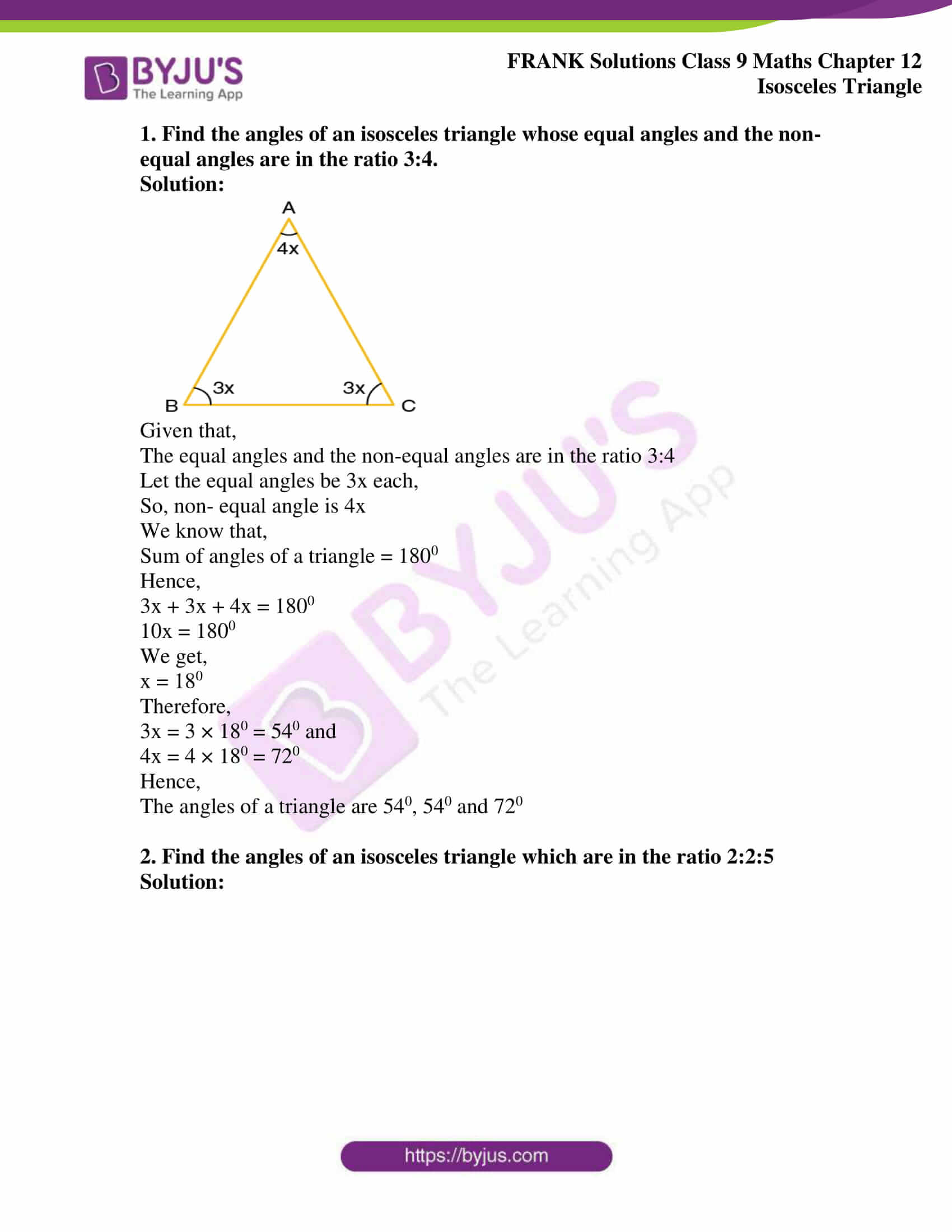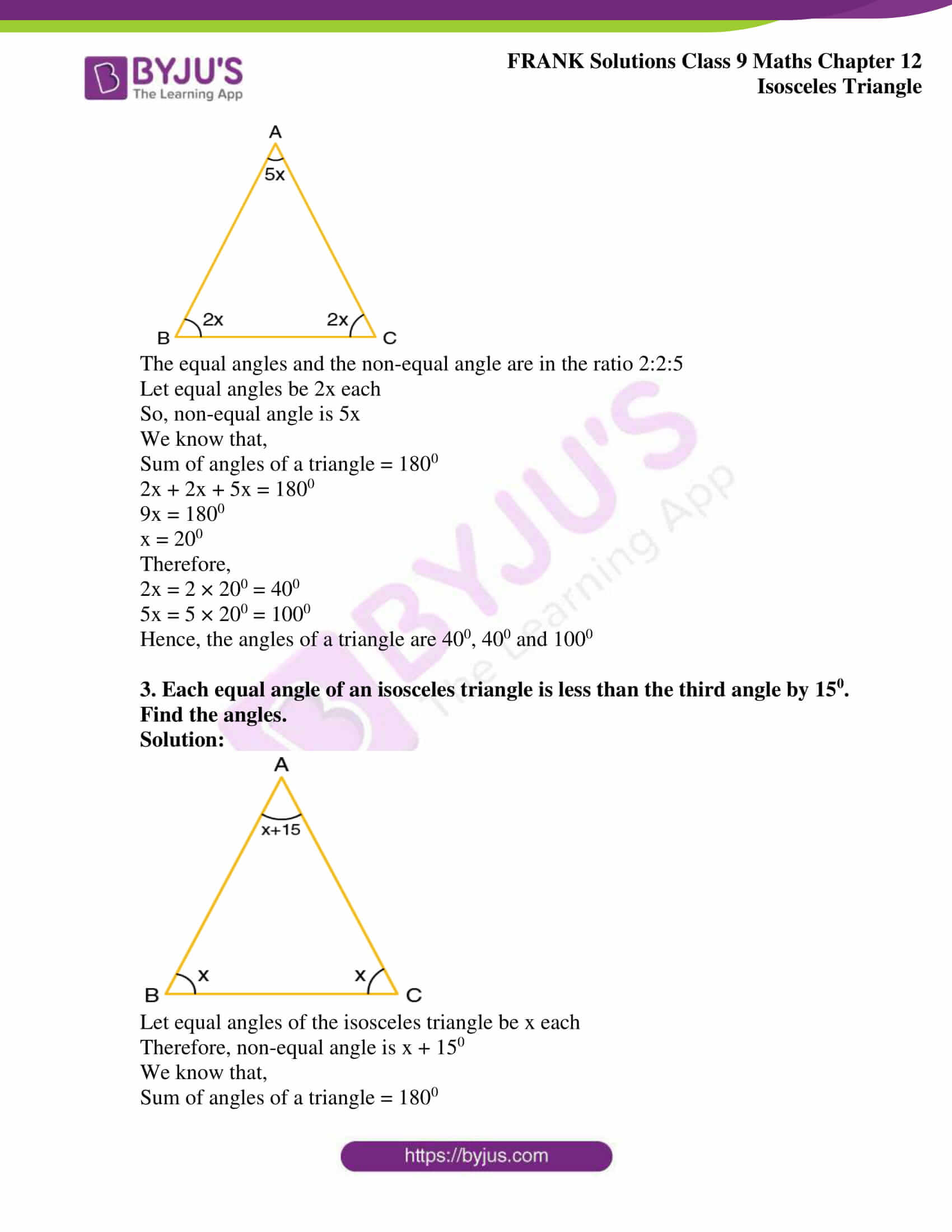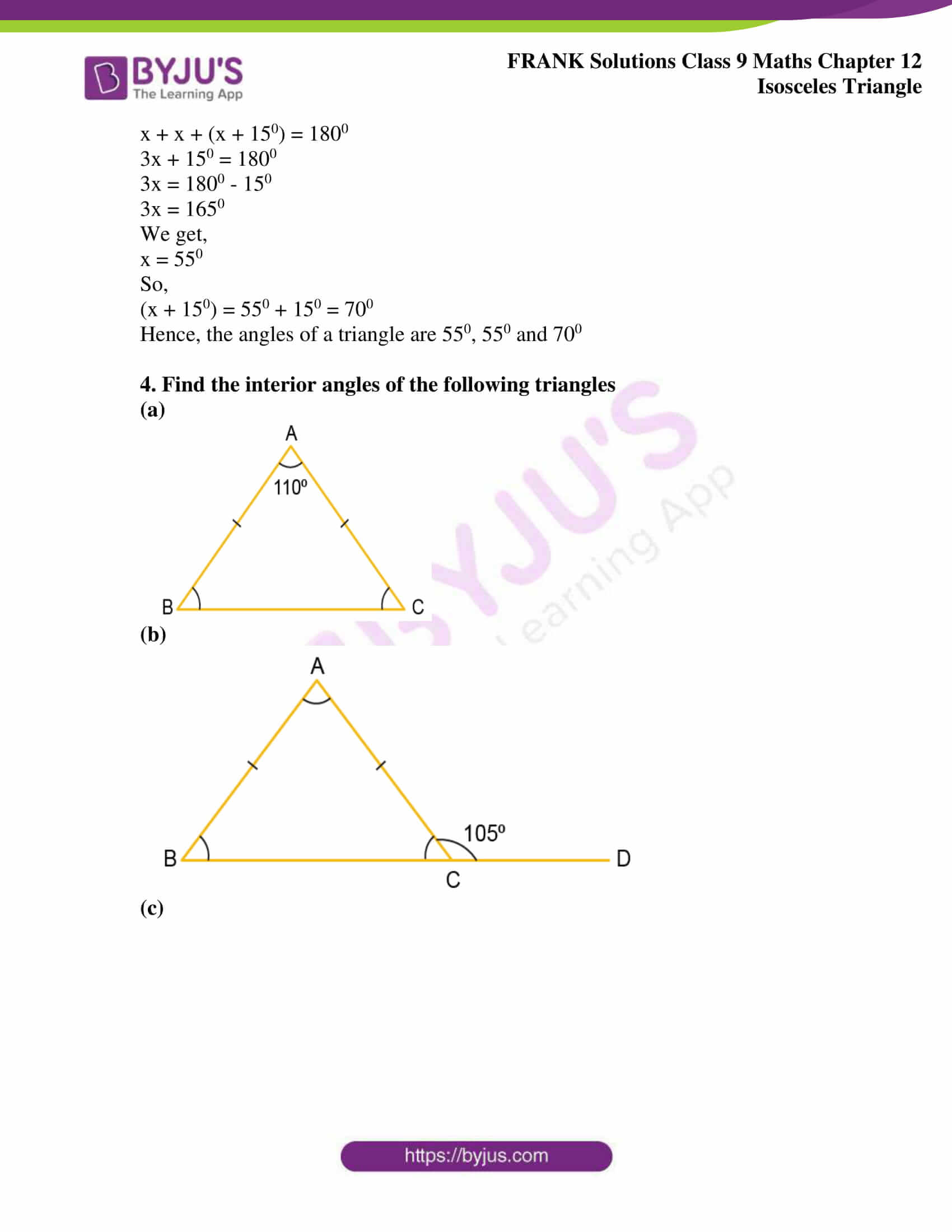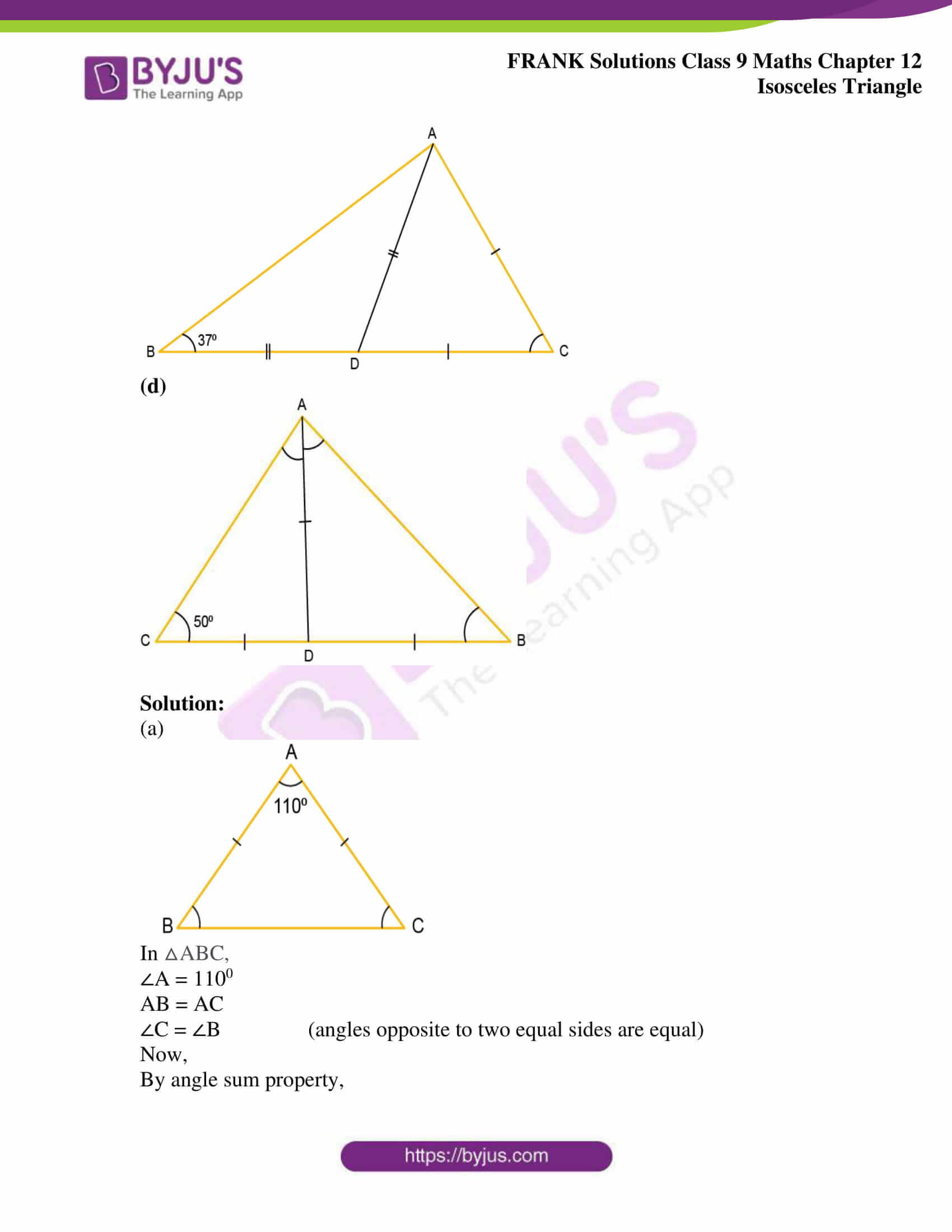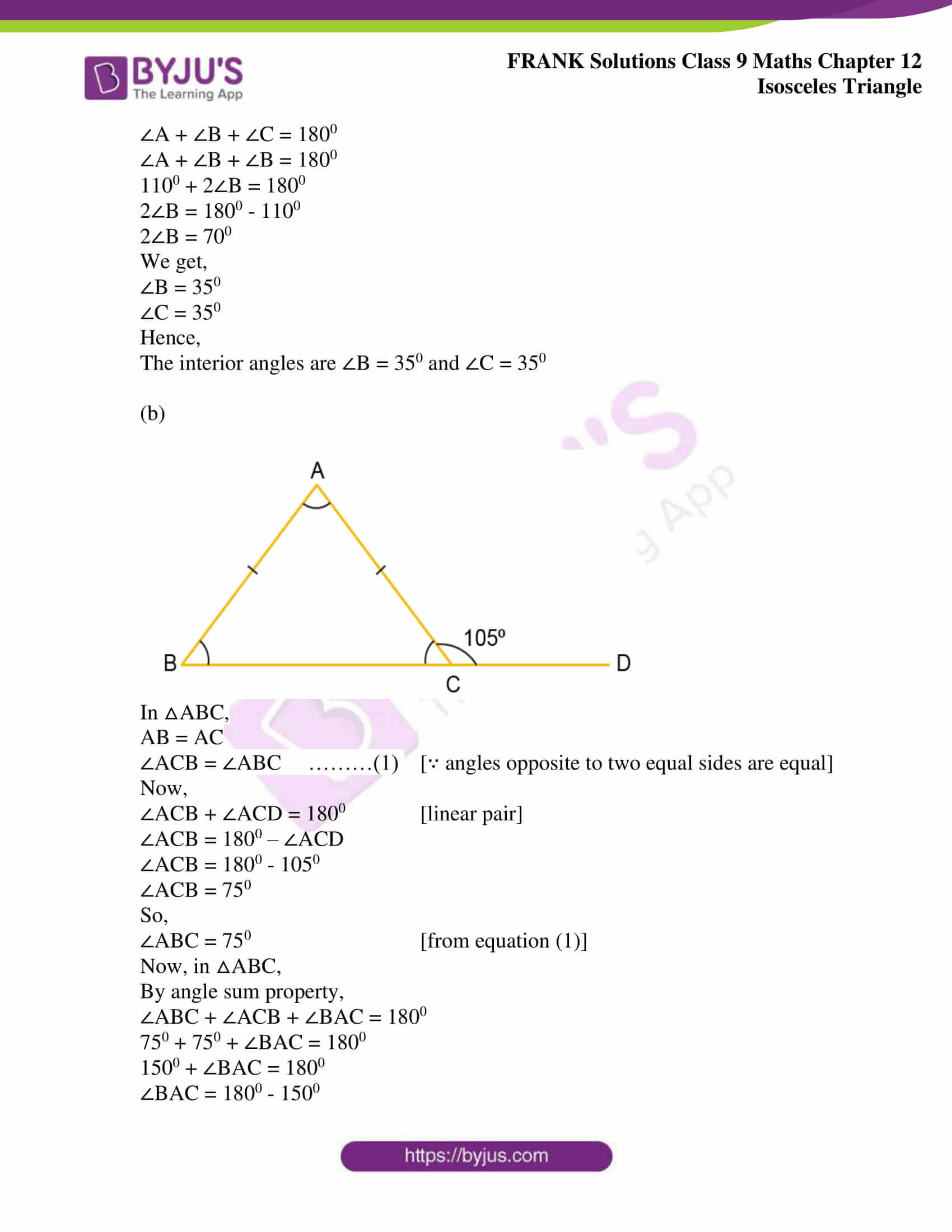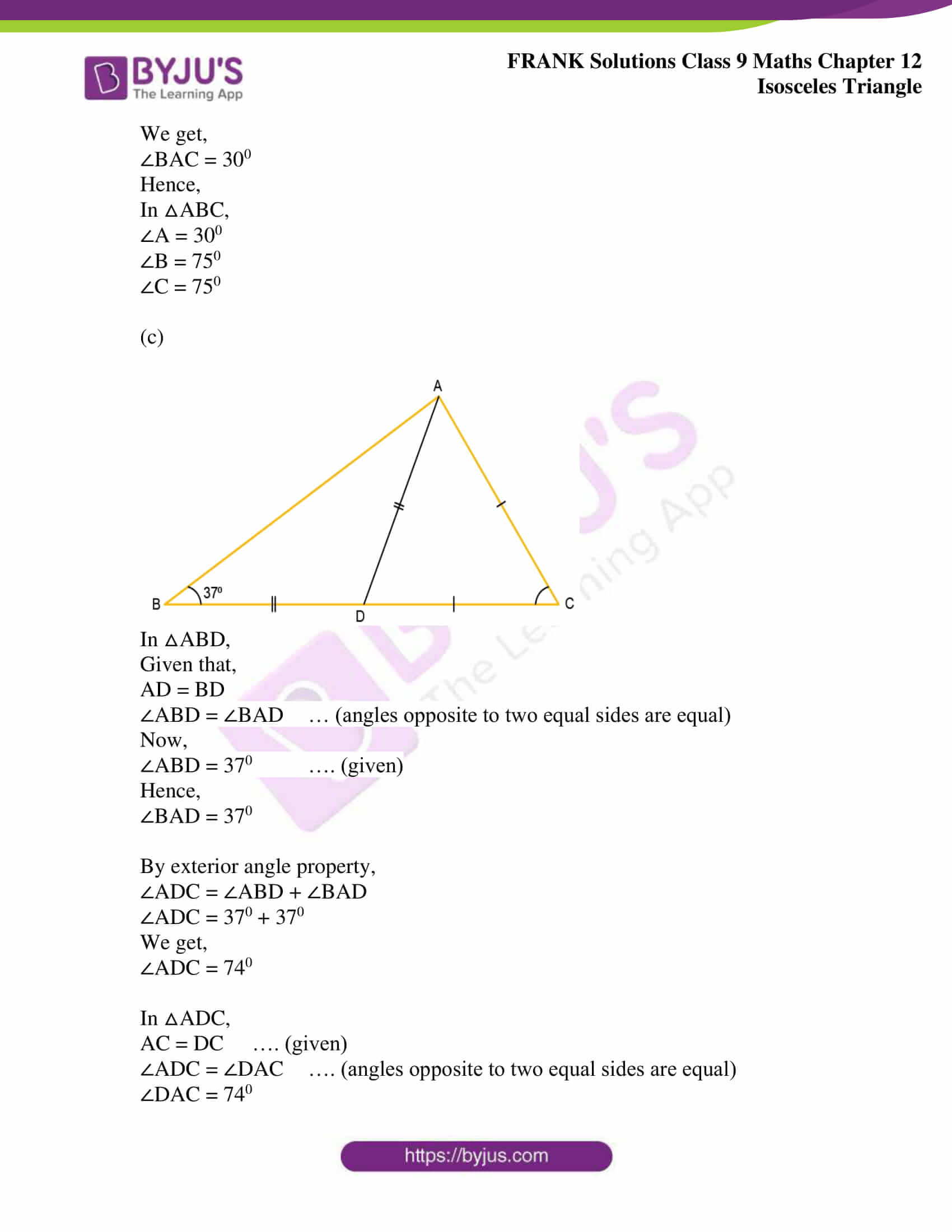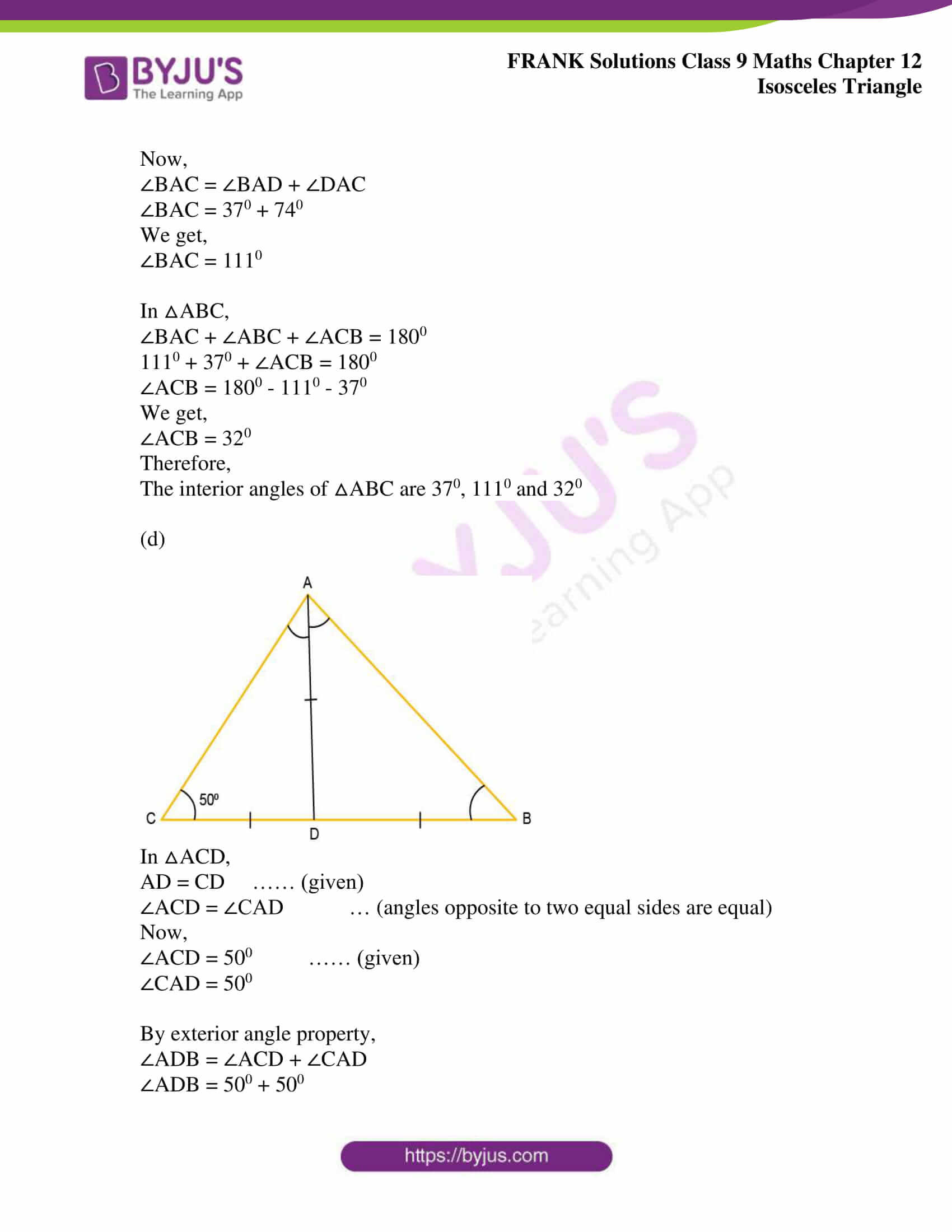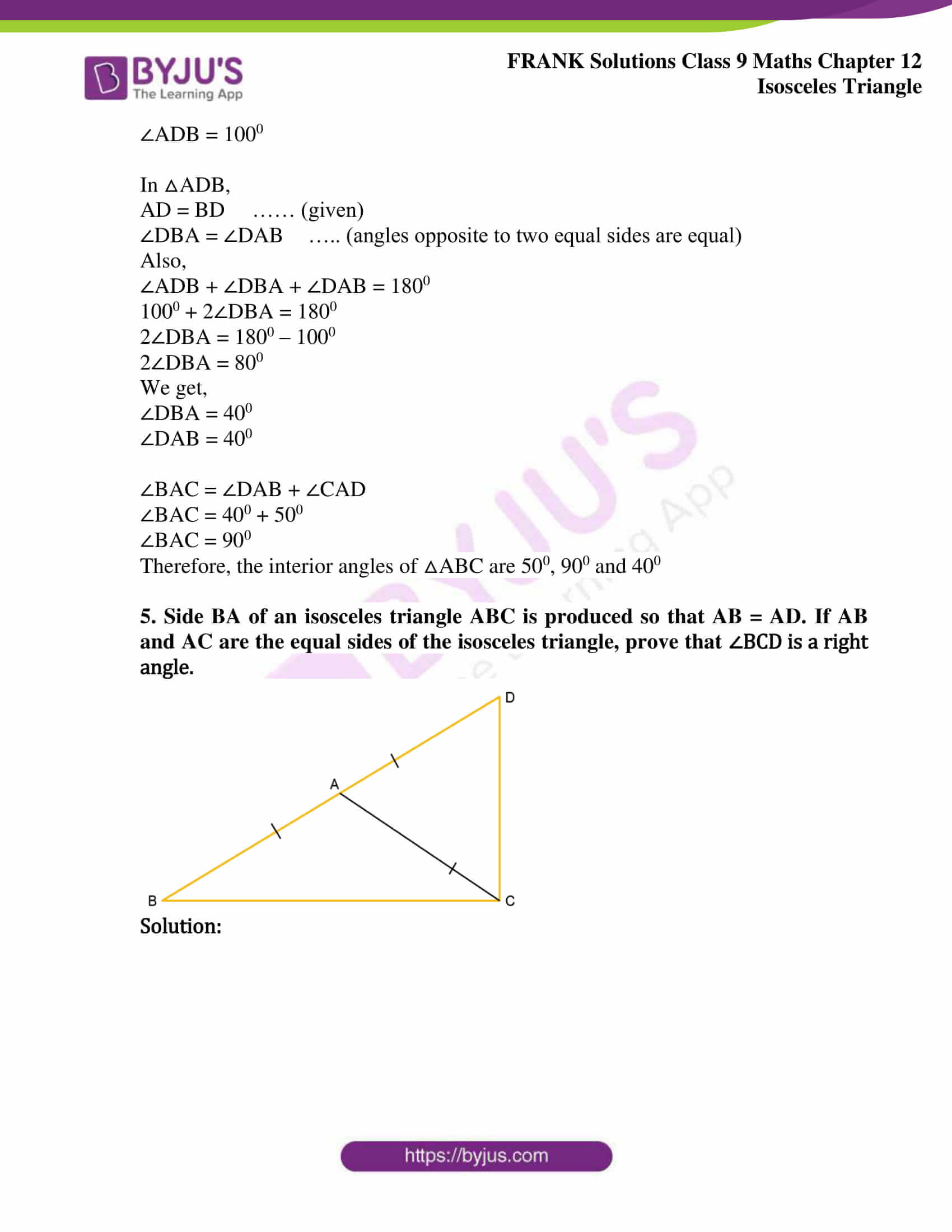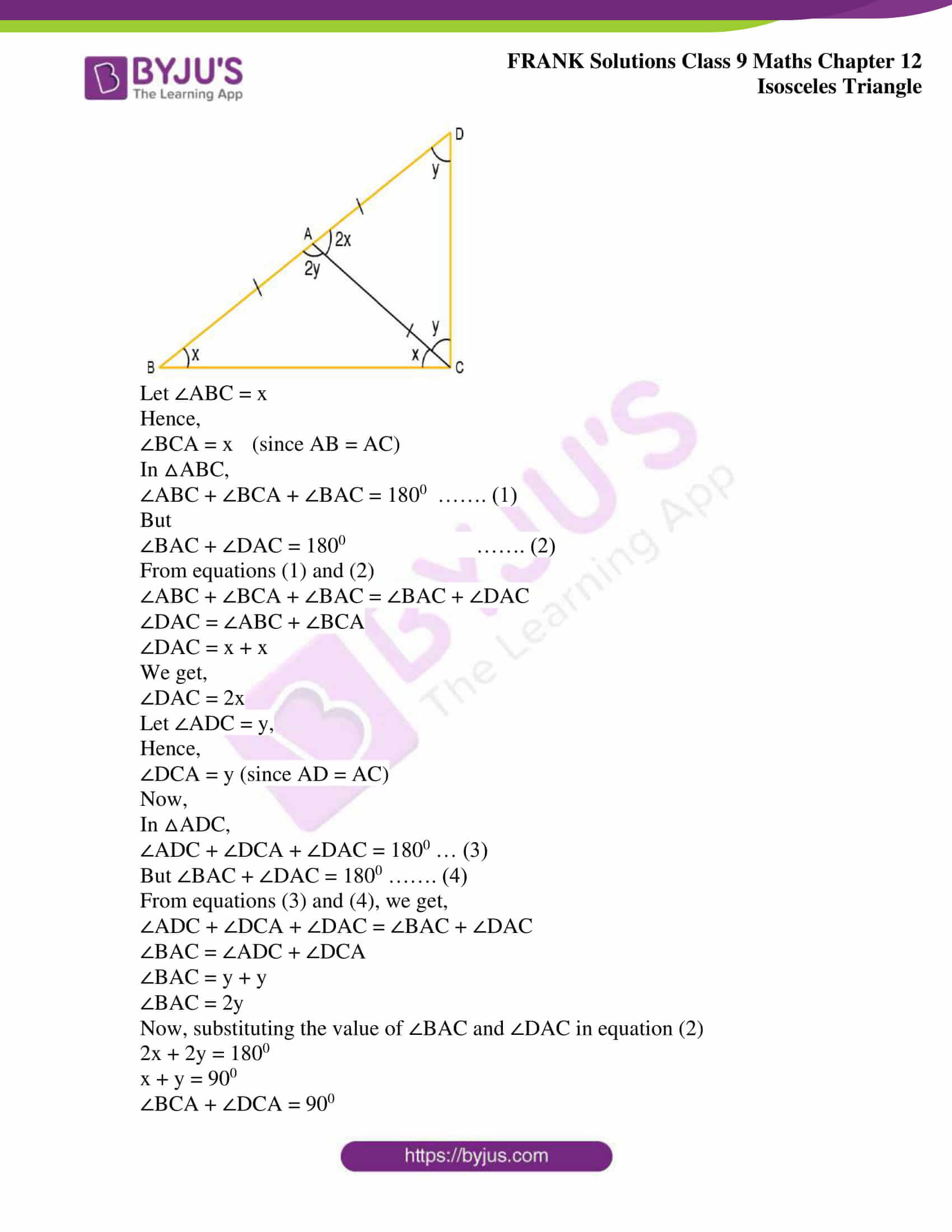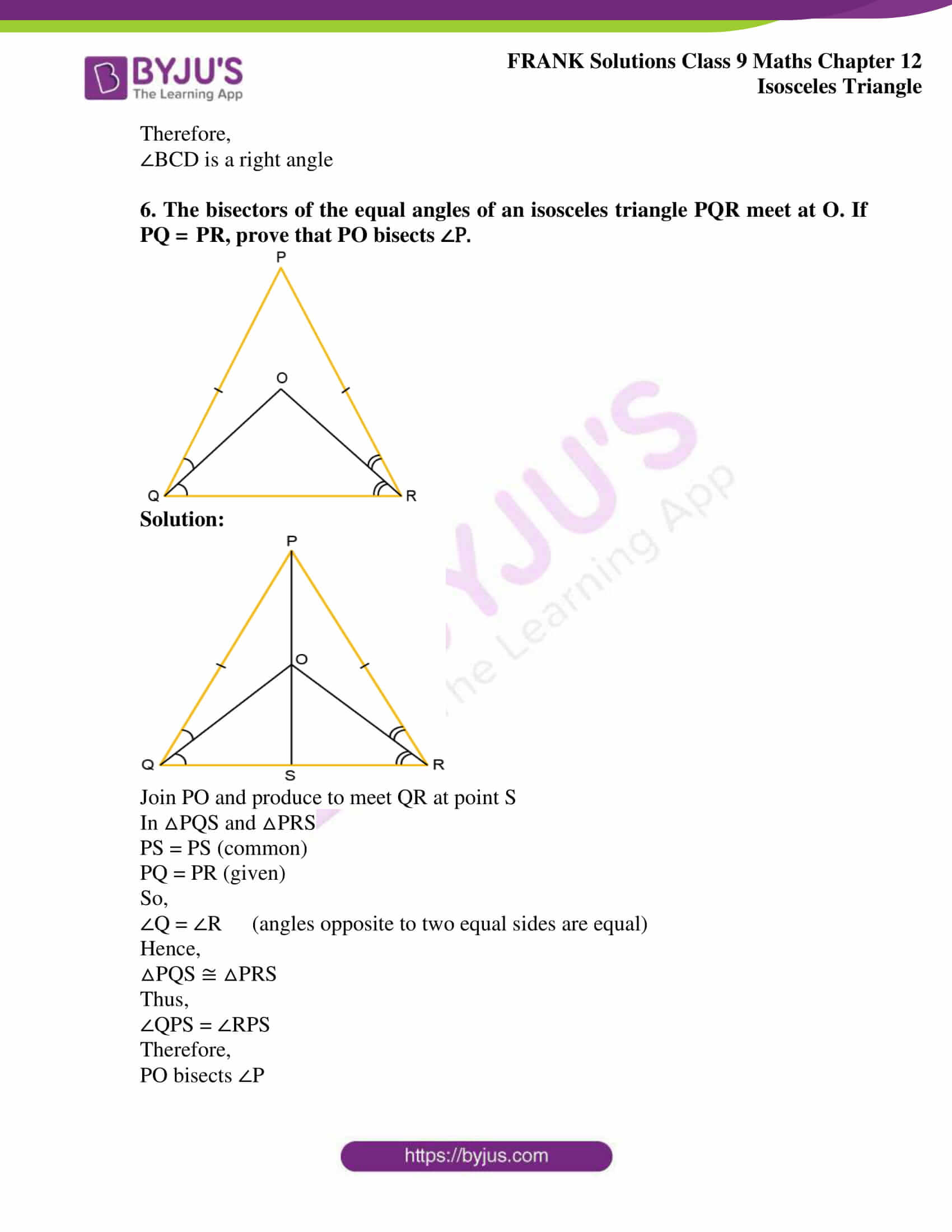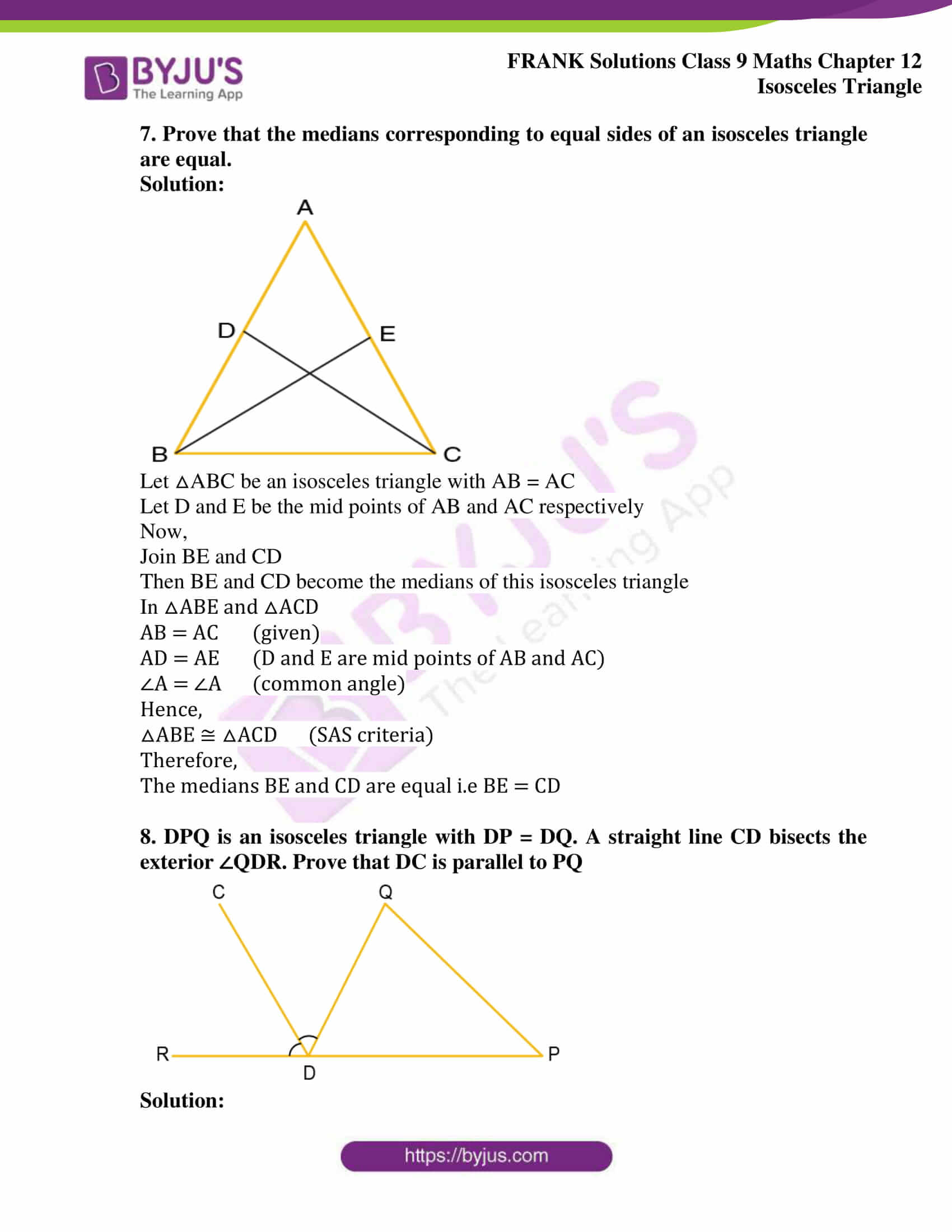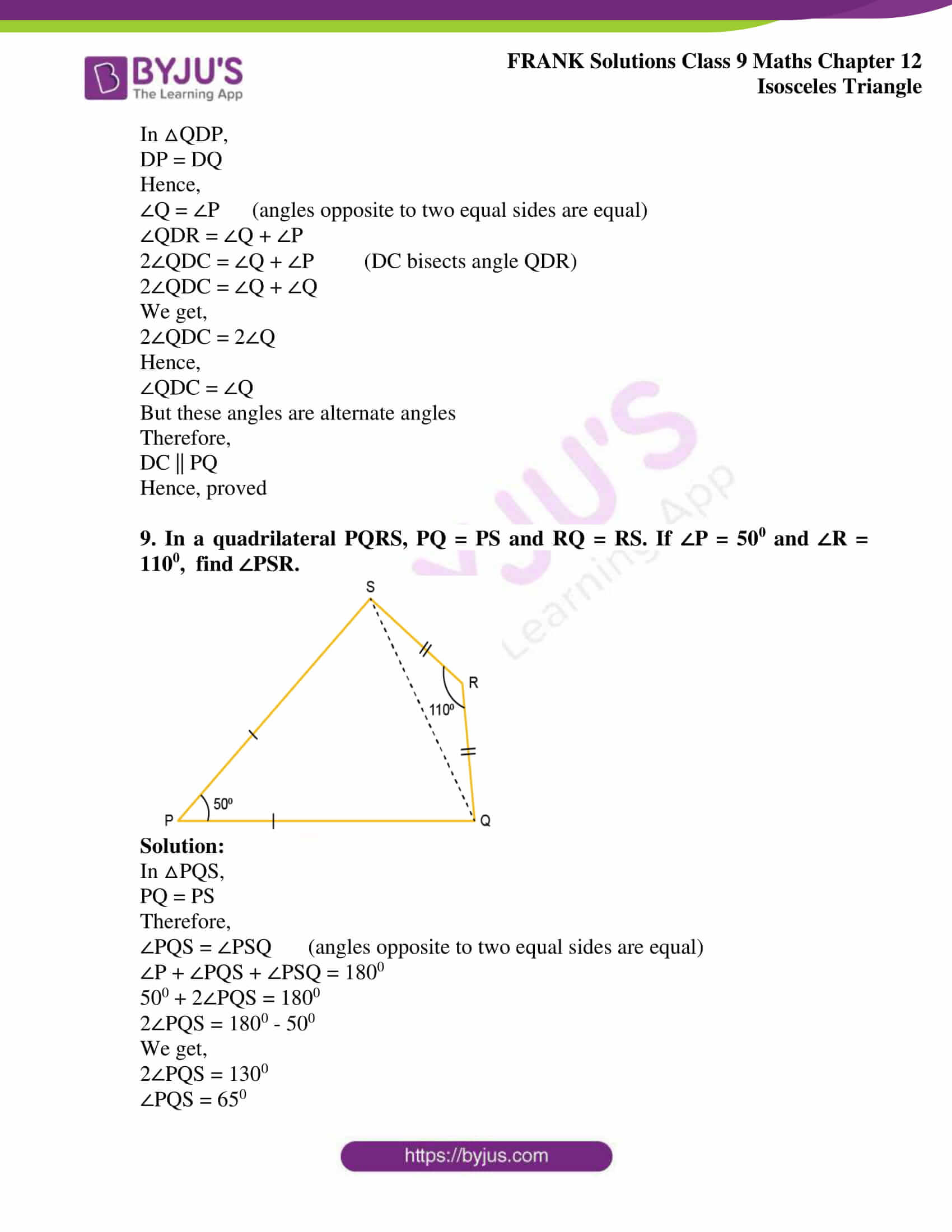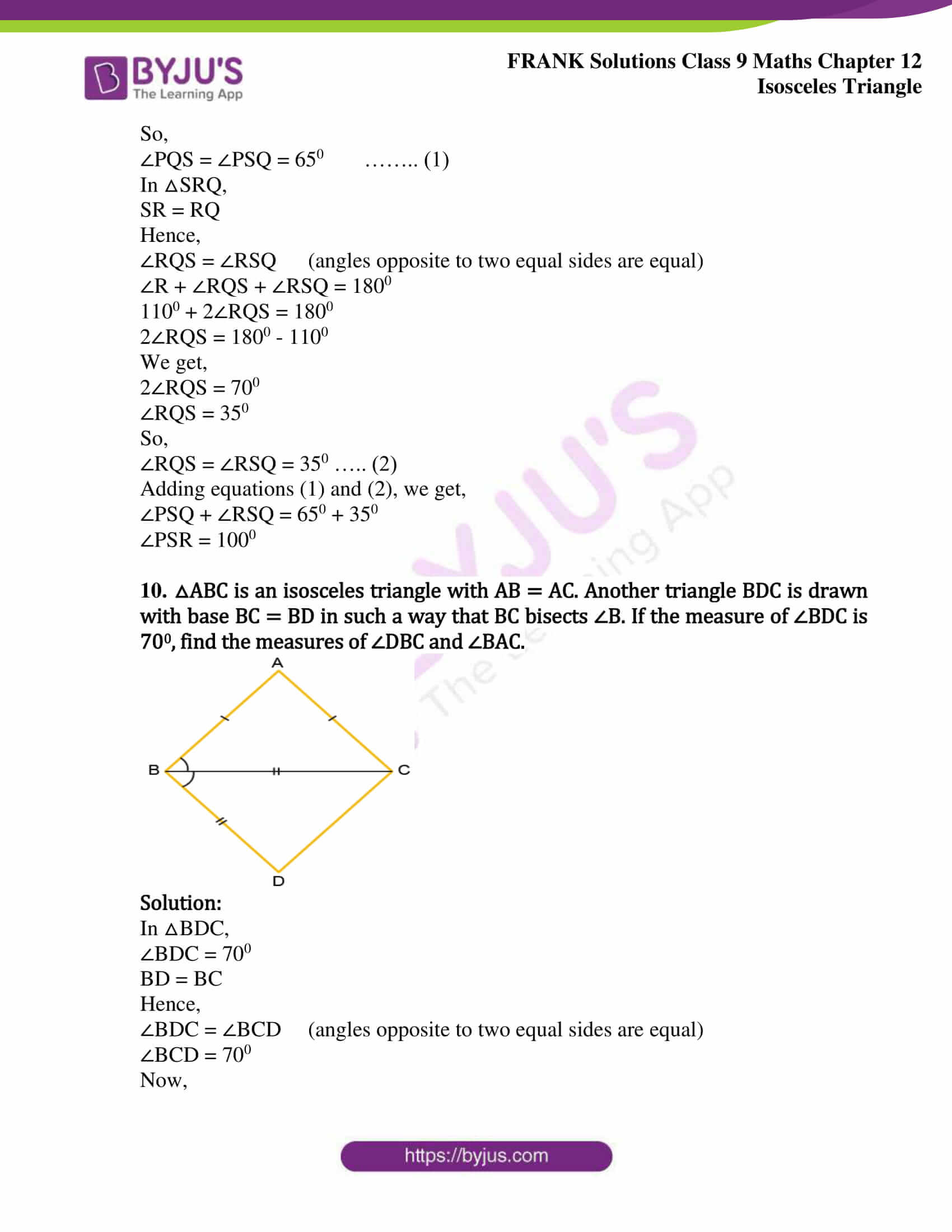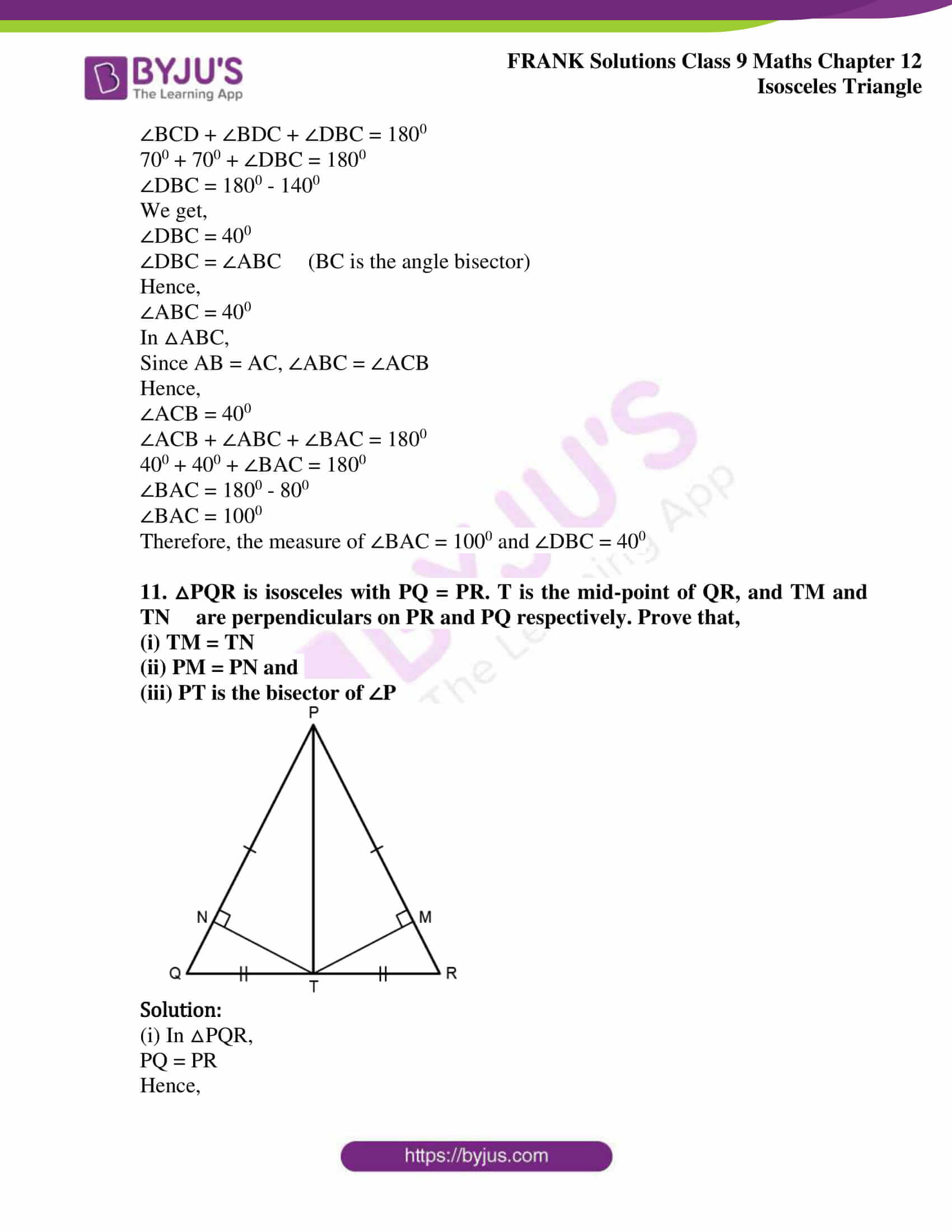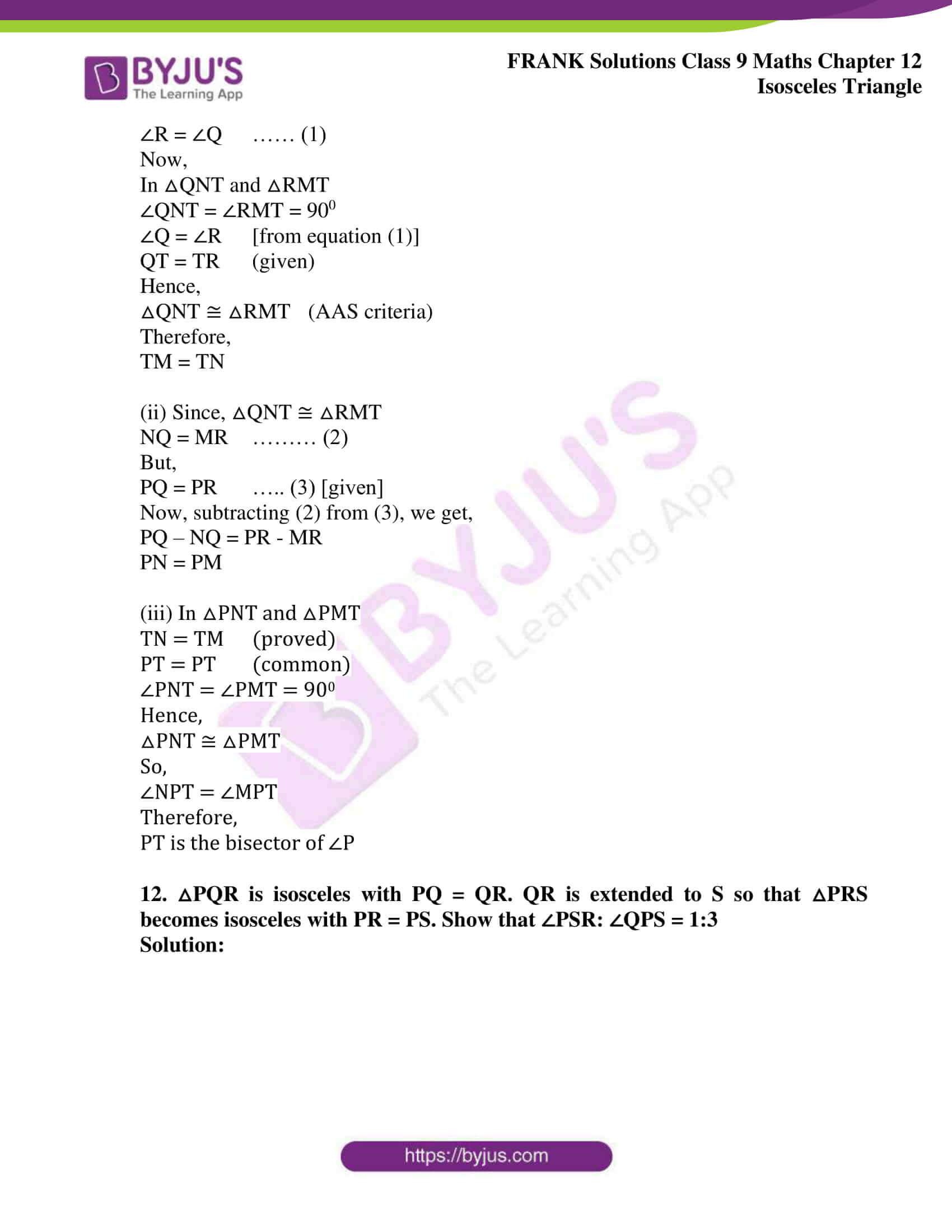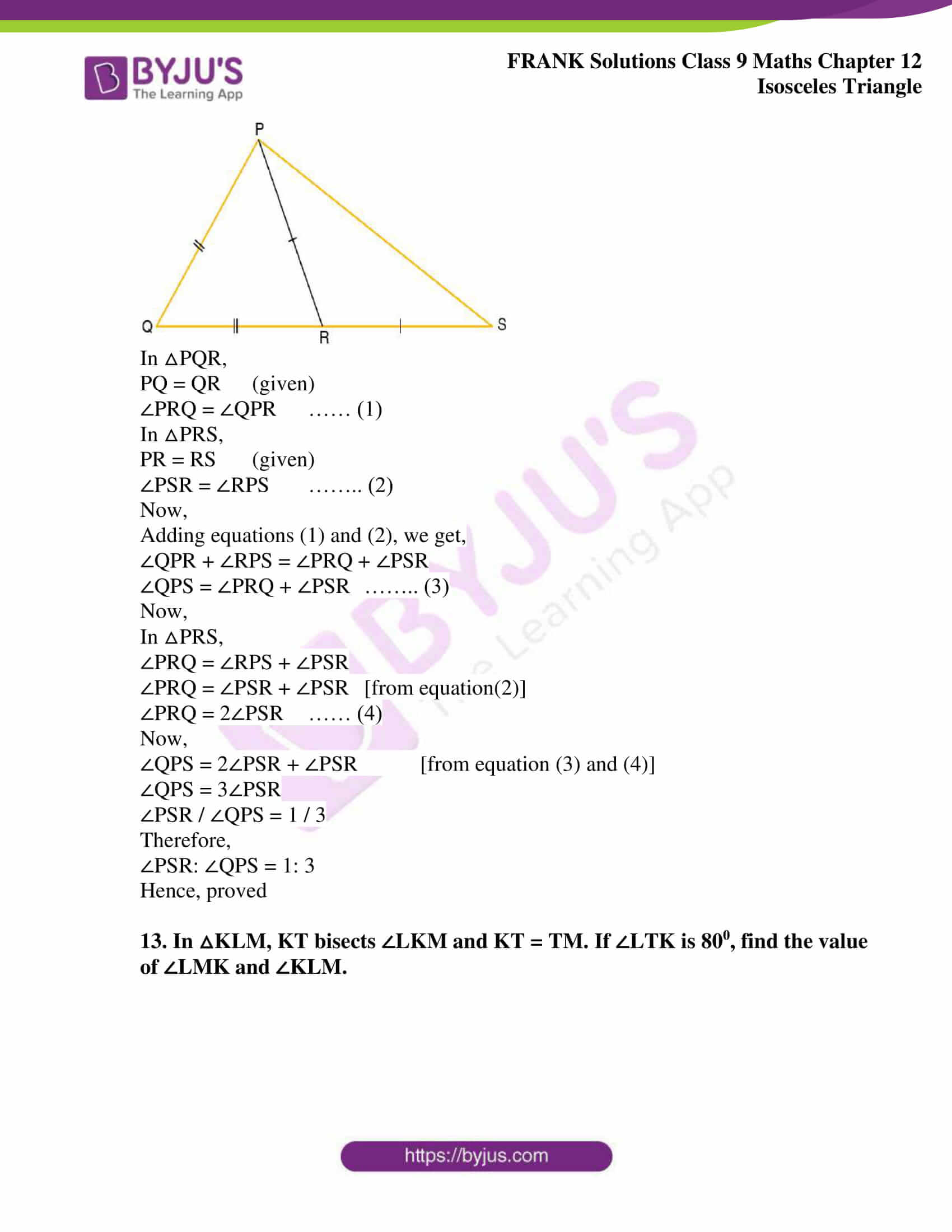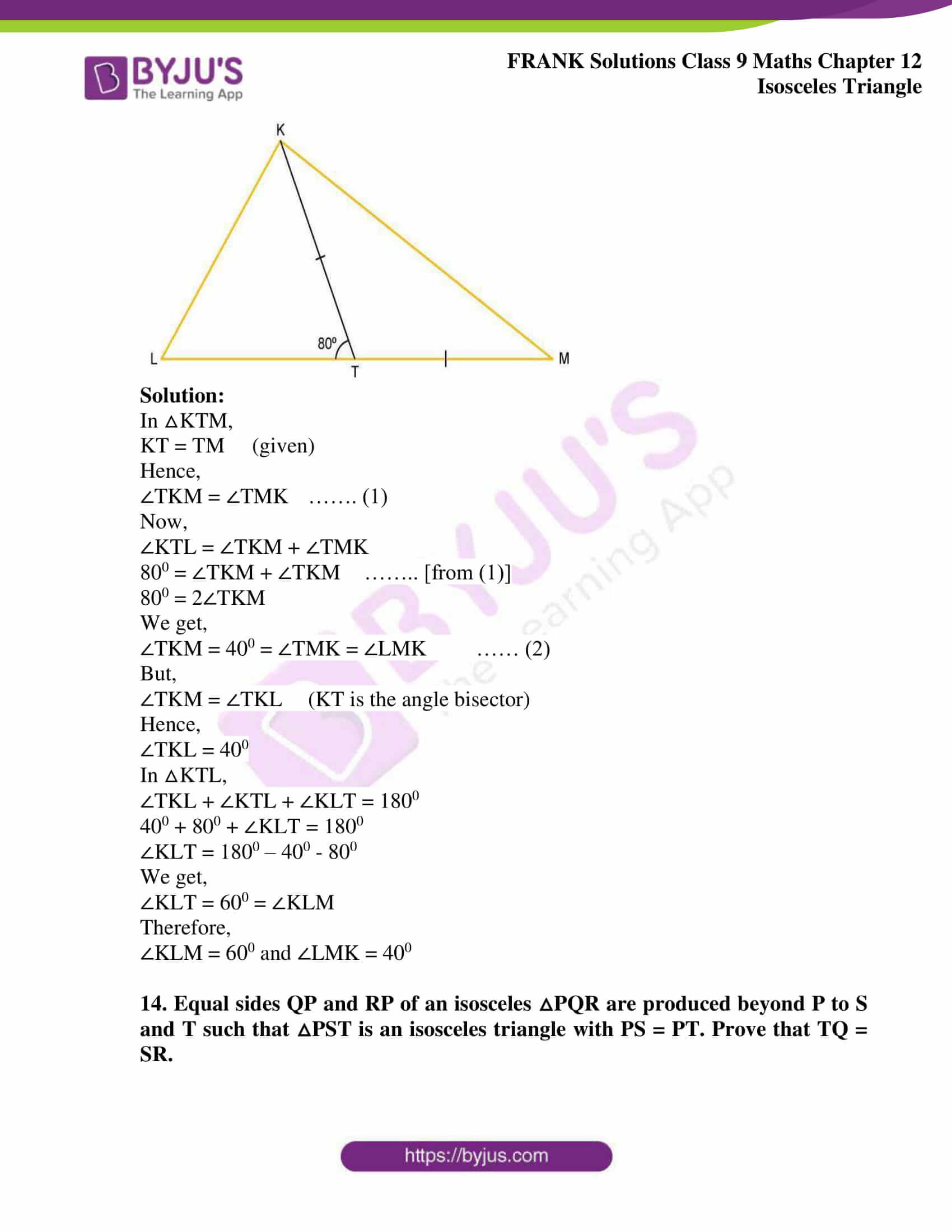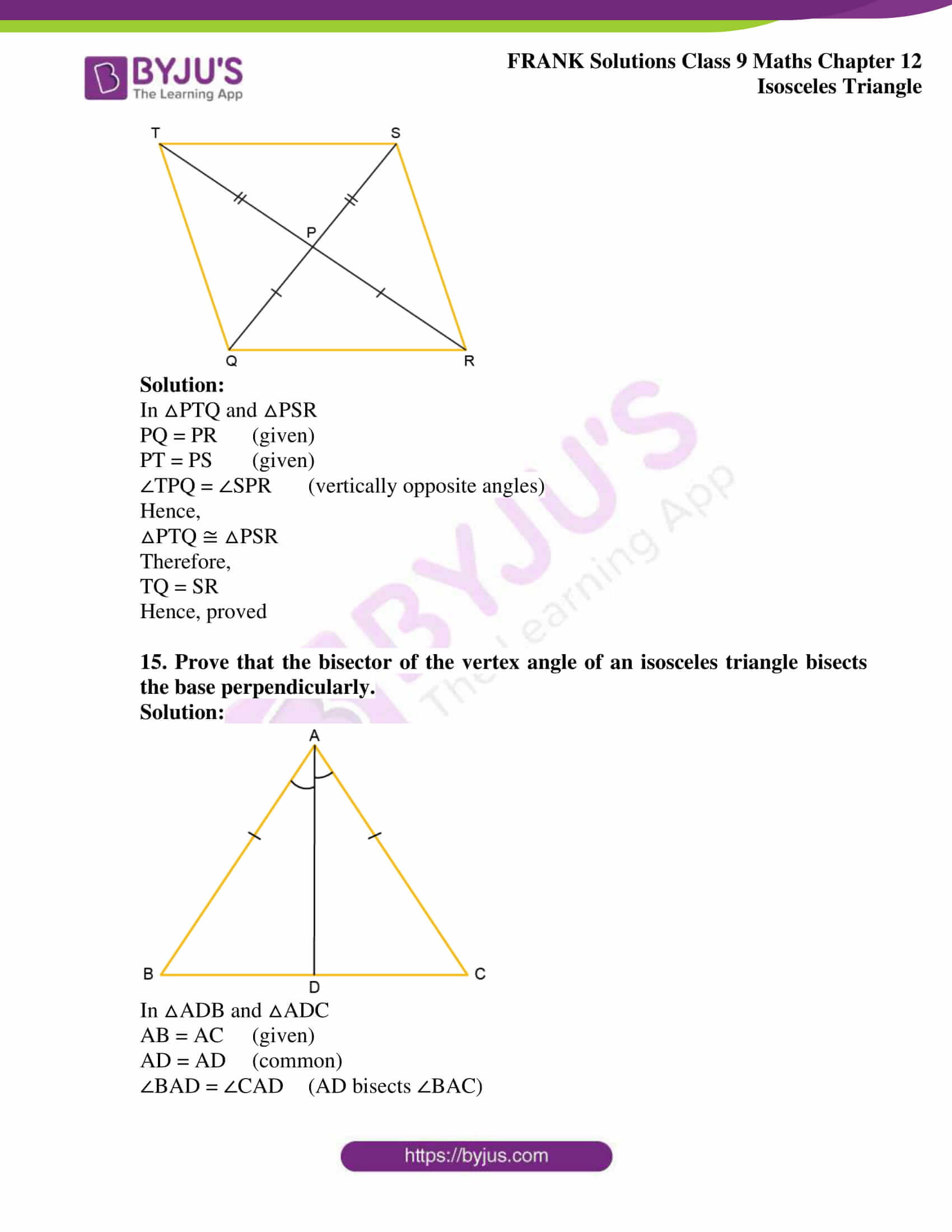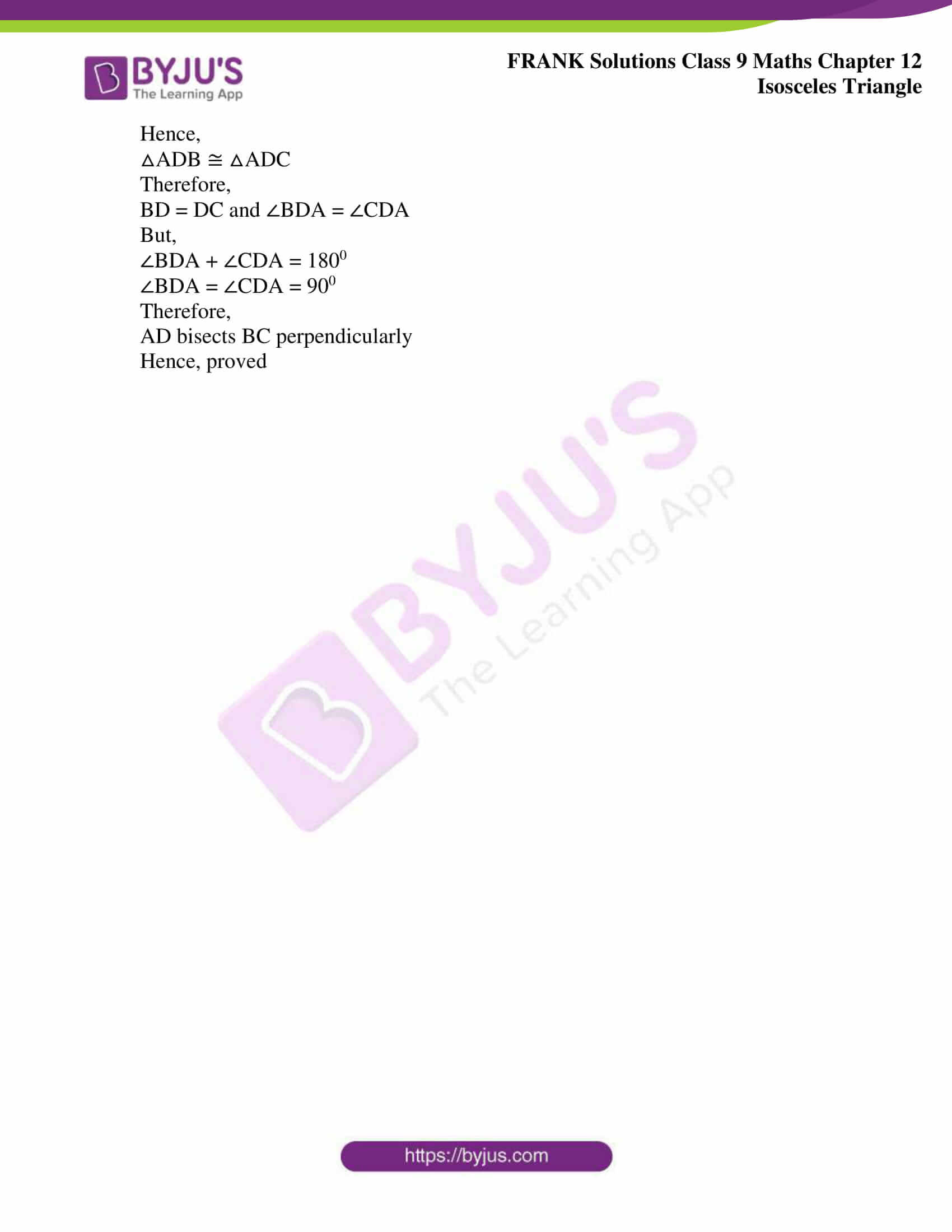## Access Frank Solutions for Class 9 Maths Chapter 12 Isosceles Triangle

1. Find the angles of an isosceles triangle whose equal angles and the non-equal angles are in the ratio 3:4.

Solution: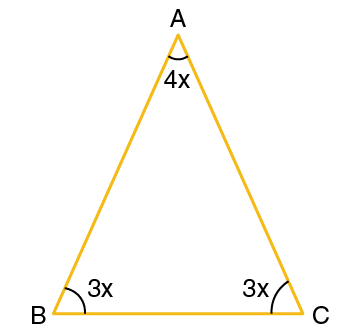Given that,

The equal angles and the non-equal angles are in the ratio 3:4

Let the equal angles be 3x each,

So, non- equal angle is 4x

We know that,

The sum of angles of a triangle = 1800

Hence,

3x + 3x + 4x = 1800

10x = 1800

We get,

x = 180

Therefore,

3x = 3 × 180 = 540 and

4x = 4 × 180 = 720

Hence,

The angles of a triangle are 540, 540 and 720

2. Find the angles of an isosceles triangle which are in the ratio 2:2:5

Solution: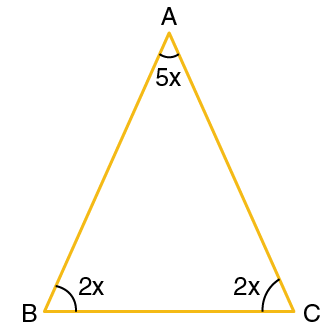The equal angles and the non-equal angle are in the ratio 2:2:5

Let equal angles be 2x each

So, the non-equal angle is 5x

We know that,

The sum of angles of a triangle = 1800

2x + 2x + 5x = 1800

9x = 1800

x = 200

Therefore,

2x = 2 × 200 = 400

5x = 5 × 200 = 1000

Hence, the angles of a triangle are 400, 400 and 1000

3. Each equal angle of an isosceles triangle is less than the third angle by 150. Find the angles.

Solution: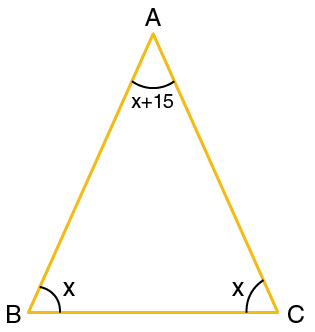Let equal angles of the isosceles triangle be x each

Therefore, the non-equal angle is x + 150

We know that,

The sum of angles of a triangle = 1800

x + x + (x + 150) = 1800

3x + 150 = 1800

3x = 1800 – 150

3x = 1650

We get,

x = 550

So,

(x + 150) = 550 + 150 = 700

Hence, the angles of a triangle are 550, 550 and 700

4. Find the interior angles of the following triangles

(a)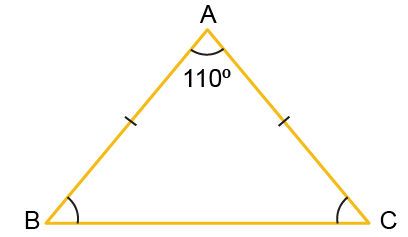(b)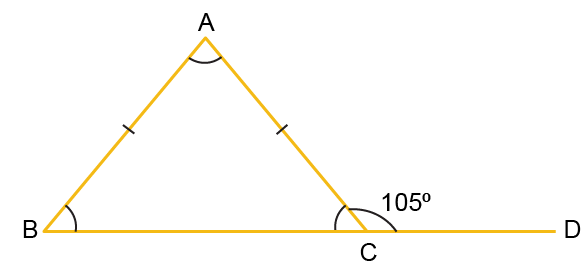(c)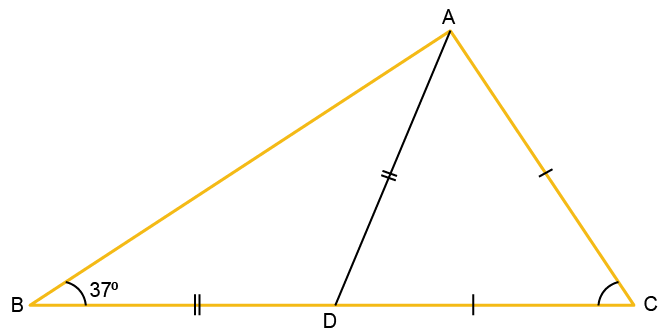(d)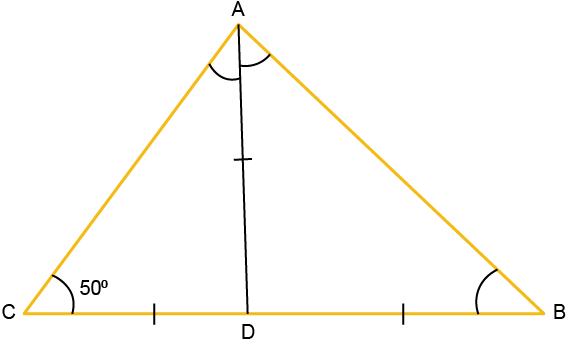Solution:

(a)In △ABC,

∠A = 1100

AB = AC

∠C = ∠B (angles opposite to two equal sides are equal)

Now,

By angle sum property,

∠A + ∠B + ∠C = 1800

∠A + ∠B + ∠B = 1800

1100 + 2∠B = 1800

2∠B = 1800 – 1100

2∠B = 700

We get,

∠B = 350

∠C = 350

Hence,

The interior angles are ∠B = 350 and ∠C = 350

(b)In △ABC,

AB = AC

∠ACB = ∠ABC ………(1) [∵ angles opposite to two equal sides are equal]

Now,

∠ACB + ∠ACD = 1800 [linear pair]

∠ACB = 1800 – ∠ACD

∠ACB = 1800 – 1050

∠ACB = 750

So,

∠ABC = 750 [from equation (1)]

Now, in △ABC,

By angle sum property,

∠ABC + ∠ACB + ∠BAC = 1800

750 + 750 + ∠BAC = 1800

1500 + ∠BAC = 1800

∠BAC = 1800 – 1500

We get,

∠BAC = 300

Hence,

In △ABC,

∠A = 300

∠B = 750

∠C = 750

(c)In △ABD,

Given that,

∠ABD = ∠BAD … (angles opposite to two equal sides are equal)

Now,

∠ABD = 370 …. (given)

Hence,

By exterior angle property,

We get,

AC = DC …. (given)

∠ADC = ∠DAC …. (angles opposite to two equal sides are equal)

∠DAC = 740

Now,

∠BAC = 370 + 740

We get,

∠BAC = 1110

In △ABC,

∠BAC + ∠ABC + ∠ACB = 1800

1110 + 370 + ∠ACB = 1800

∠ACB = 1800 – 1110 – 370

We get,

∠ACB = 320

Therefore,

The interior angles of △ABC are 370, 1110 and 320

(d)In △ACD,

∠ACD = ∠CAD … (angles opposite to two equal sides are equal)

Now,

∠ACD = 500 …… (given)

By exterior angle property,

∠DBA = ∠DAB ….. (angles opposite to two equal sides are equal)

Also,

∠ADB + ∠DBA + ∠DAB = 1800

1000 + 2∠DBA = 1800

2∠DBA = 1800 – 1000

2∠DBA = 800

We get,

∠DBA = 400

∠DAB = 400

∠BAC = 400 + 500

∠BAC = 900

Therefore, the interior angles of △ABC are 500, 900 and 400

5. Side BA of an isosceles triangle ABC is produced so that AB = AD. If AB and AC are the equal sides of the isosceles triangle, prove that ∠BCD is a right angle.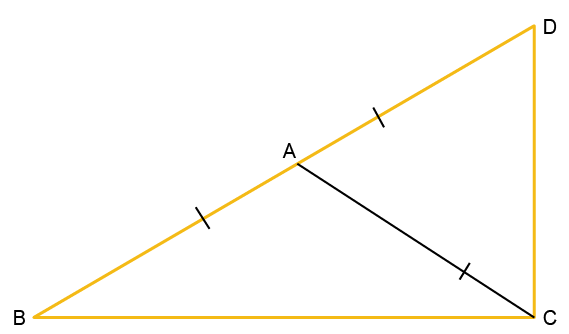Solution: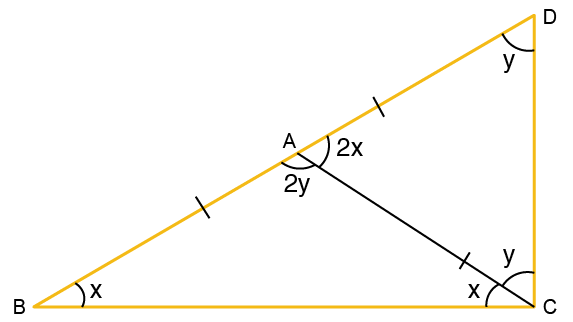Let ∠ABC = x

Hence,

∠BCA = x (since AB = AC)

In △ABC,

∠ABC + ∠BCA + ∠BAC = 1800 ……. (1)

But

∠BAC + ∠DAC = 1800 ……. (2)

From equations (1) and (2)

∠ABC + ∠BCA + ∠BAC = ∠BAC + ∠DAC

∠DAC = ∠ABC + ∠BCA

∠DAC = x + x

We get,

∠DAC = 2x

Hence,

∠DCA = y (since AD = AC)

Now,

∠ADC + ∠DCA + ∠DAC = 1800 … (3)

But ∠BAC + ∠DAC = 1800 ……. (4)

From equations (3) and (4), we get,

∠ADC + ∠DCA + ∠DAC = ∠BAC + ∠DAC

∠BAC = y + y

∠BAC = 2y

Now, substituting the value of ∠BAC and ∠DAC in equation (2)

2x + 2y = 1800

x + y = 900

∠BCA + ∠DCA = 900

Therefore,

∠BCD is a right angle

6. The bisectors of the equal angles of an isosceles triangle PQR meet at O. If PQ = PR, prove that PO bisects ∠P.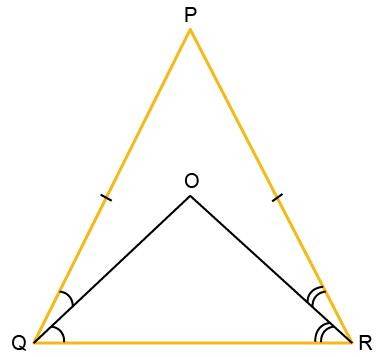Solution: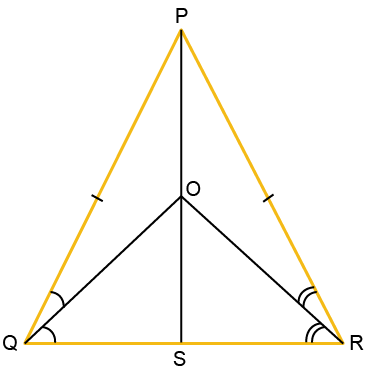Join PO and produce to meet QR at point S

In △PQS and △PRS

PS = PS (common)

PQ = PR (given)

So,

∠Q = ∠R (angles opposite to two equal sides are equal)

Hence,

△PQS ≅ △PRS

Thus,

∠QPS = ∠RPS

Therefore,

PO bisects ∠P

7. Prove that the medians corresponding to equal sides of an isosceles triangle are equal.

Solution: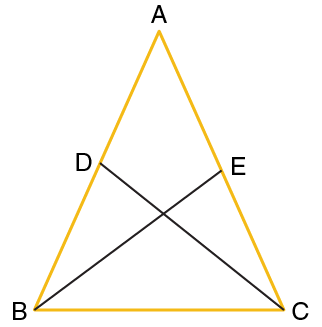Let △ABC be an isosceles triangle with AB = AC

Let D and E be the midpoints of AB and AC, respectively

Now,

Join BE and CD

Then BE and CD become the medians of this isosceles triangle

In △ABE and △ACD

AB = AC (given)

AD = AE (D and E are midpoints of AB and AC)

∠A = ∠A (common angle)

Hence,

△ABE ≅ △ACD (SAS criteria)

Therefore,

The medians BE and CD are equal, i.e., BE = CD

8. DPQ is an isosceles triangle with DP = DQ. A straight line CD bisects the exterior ∠QDR. Prove that DC is parallel to PQ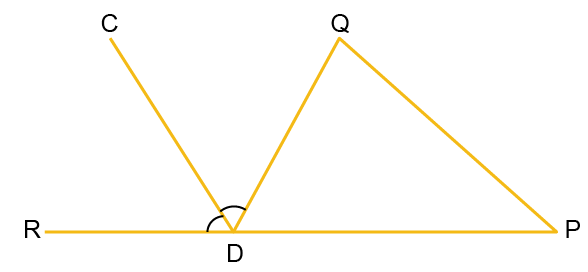Solution:

In △QDP,

DP = DQ

Hence,

∠Q = ∠P (angles opposite to two equal sides are equal)

∠QDR = ∠Q + ∠P

2∠QDC = ∠Q + ∠P (DC bisects angle QDR)

2∠QDC = ∠Q + ∠Q

We get,

2∠QDC = 2∠Q

Hence,

∠QDC = ∠Q

But these angles are alternate angles

Therefore,

DC || PQ

Hence, proved

9. In a quadrilateral PQRS, PQ = PS and RQ = RS. If ∠P = 500 and ∠R = 1100, find ∠PSR.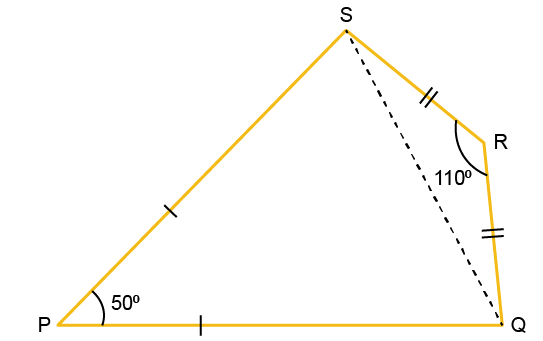Solution:

In △PQS,

PQ = PS

Therefore,

∠PQS = ∠PSQ (angles opposite to two equal sides are equal)

∠P + ∠PQS + ∠PSQ = 1800

500 + 2∠PQS = 1800

2∠PQS = 1800 – 500

We get,

2∠PQS = 1300

∠PQS = 650

So,

∠PQS = ∠PSQ = 650 …….. (1)

In △SRQ,

SR = RQ

Hence,

∠RQS = ∠RSQ (angles opposite to two equal sides are equal)

∠R + ∠RQS + ∠RSQ = 1800

1100 + 2∠RQS = 1800

2∠RQS = 1800 – 1100

We get,

2∠RQS = 700

∠RQS = 350

So,

∠RQS = ∠RSQ = 350 ….. (2)

Adding equations (1) and (2), we get,

∠PSQ + ∠RSQ = 650 + 350

∠PSR = 1000

10. △ABC is an isosceles triangle with AB = AC. Another triangle, BDC is drawn with base BC = BD in such a way that BC bisects ∠B. If the measure of ∠BDC is 700, find the measures of ∠DBC and ∠BAC.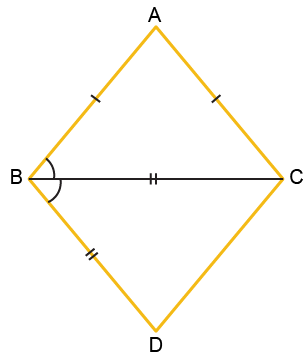Solution:

In △BDC,

∠BDC = 700

BD = BC

Hence,

∠BDC = ∠BCD (angles opposite to two equal sides are equal)

∠BCD = 700

Now,

∠BCD + ∠BDC + ∠DBC = 1800

700 + 700 + ∠DBC = 1800

∠DBC = 1800 – 1400

We get,

∠DBC = 400

∠DBC = ∠ABC (BC is the angle bisector)

Hence,

∠ABC = 400

In △ABC,

Since AB = AC, ∠ABC = ∠ACB

Hence,

∠ACB = 400

∠ACB + ∠ABC + ∠BAC = 1800

400 + 400 + ∠BAC = 1800

∠BAC = 1800 – 800

∠BAC = 1000

Therefore, the measure of ∠BAC = 1000 and ∠DBC = 400

11. △PQR is isosceles with PQ = PR. T is the mid-point of QR, and TM and TN are perpendiculars on PR and PQ, respectively. Prove that,

(i) TM = TN

(ii) PM = PN and

(iii) PT is the bisector of ∠P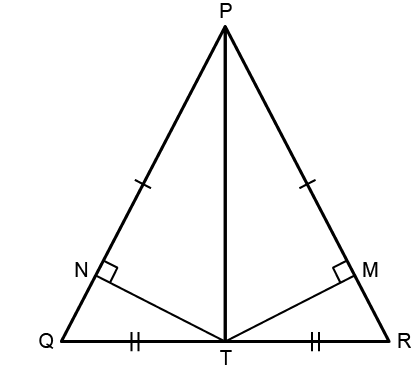Solution:

(i) In △PQR,

PQ = PR

Hence,

∠R = ∠Q …… (1)

Now,

In △QNT and △RMT

∠QNT = ∠RMT = 900

∠Q = ∠R [from equation (1)]

QT = TR (given)

Hence,

△QNT ≅ △RMT (AAS criteria)

Therefore,

TM = TN

(ii) Since, △QNT ≅ △RMT

NQ = MR ……… (2)

But,

PQ = PR ….. (3) [given]

Now, subtracting (2) from (3), we get,

PQ – NQ = PR – MR

PN = PM

(iii) In △PNT and △PMT

TN = TM (proved)

PT = PT (common)

∠PNT = ∠PMT = 900

Hence,

△PNT ≅ △PMT

So,

∠NPT = ∠MPT

Therefore,

PT is the bisector of ∠P

12. △PQR is isosceles with PQ = QR. QR is extended to S so that △PRS becomes isosceles with PR = PS. Show that ∠PSR: ∠QPS = 1:3

Solution: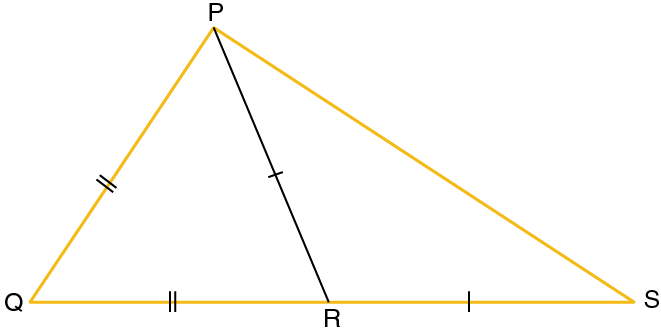In △PQR,

PQ = QR (given)

∠PRQ = ∠QPR …… (1)

In △PRS,

PR = RS (given)

∠PSR = ∠RPS …….. (2)

Now,

Adding equations (1) and (2), we get,

∠QPR + ∠RPS = ∠PRQ + ∠PSR

∠QPS = ∠PRQ + ∠PSR …….. (3)

Now,

In △PRS,

∠PRQ = ∠RPS + ∠PSR

∠PRQ = ∠PSR + ∠PSR [from equation(2)]

∠PRQ = 2∠PSR …… (4)

Now,

∠QPS = 2∠PSR + ∠PSR [from equation (3) and (4)]

∠QPS = 3∠PSR

∠PSR / ∠QPS = 1 / 3

Therefore,

∠PSR: ∠QPS = 1: 3

Hence, proved

13. In △KLM, KT bisects ∠LKM and KT = TM. If ∠LTK is 800, find the value of ∠LMK and ∠KLM.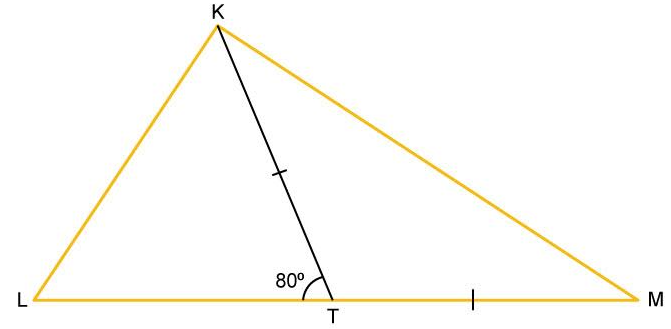Solution:

In △KTM,

KT = TM (given)

Hence,

∠TKM = ∠TMK ……. (1)

Now,

∠KTL = ∠TKM + ∠TMK

800 = ∠TKM + ∠TKM …….. [from (1)]

800 = 2∠TKM

We get,

∠TKM = 400 = ∠TMK = ∠LMK …… (2)

But,

∠TKM = ∠TKL (KT is the angle bisector)

Hence,

∠TKL = 400

In △KTL,

∠TKL + ∠KTL + ∠KLT = 1800

400 + 800 + ∠KLT = 1800

∠KLT = 1800 – 400 – 800

We get,

∠KLT = 600 = ∠KLM

Therefore,

∠KLM = 600 and ∠LMK = 400

14. Equal sides QP and RP of an isosceles △PQR are produced beyond P to S and T such that △PST is an isosceles triangle with PS = PT. Prove that TQ = SR.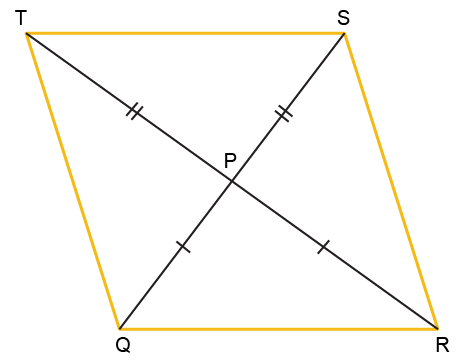Solution:

In △PTQ and △PSR

PQ = PR (given)

PT = PS (given)

∠TPQ = ∠SPR (vertically opposite angles)

Hence,

△PTQ ≅ △PSR

Therefore,

TQ = SR

Hence, proved

15. Prove that the bisector of the vertex angle of an isosceles triangle bisects the base perpendicularly.

Solution: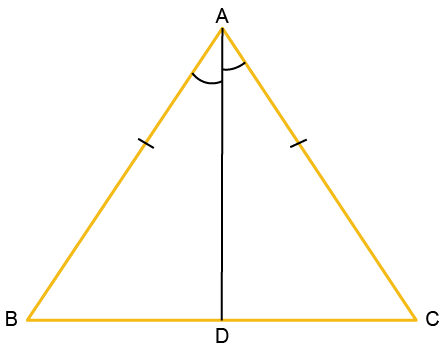AB = AC (given)

Hence,

Therefore,

BD = DC and ∠BDA = ∠CDA

But,

∠BDA + ∠CDA = 1800

∠BDA = ∠CDA = 900

Therefore,